# LOG#029. Interstellar trips in SR.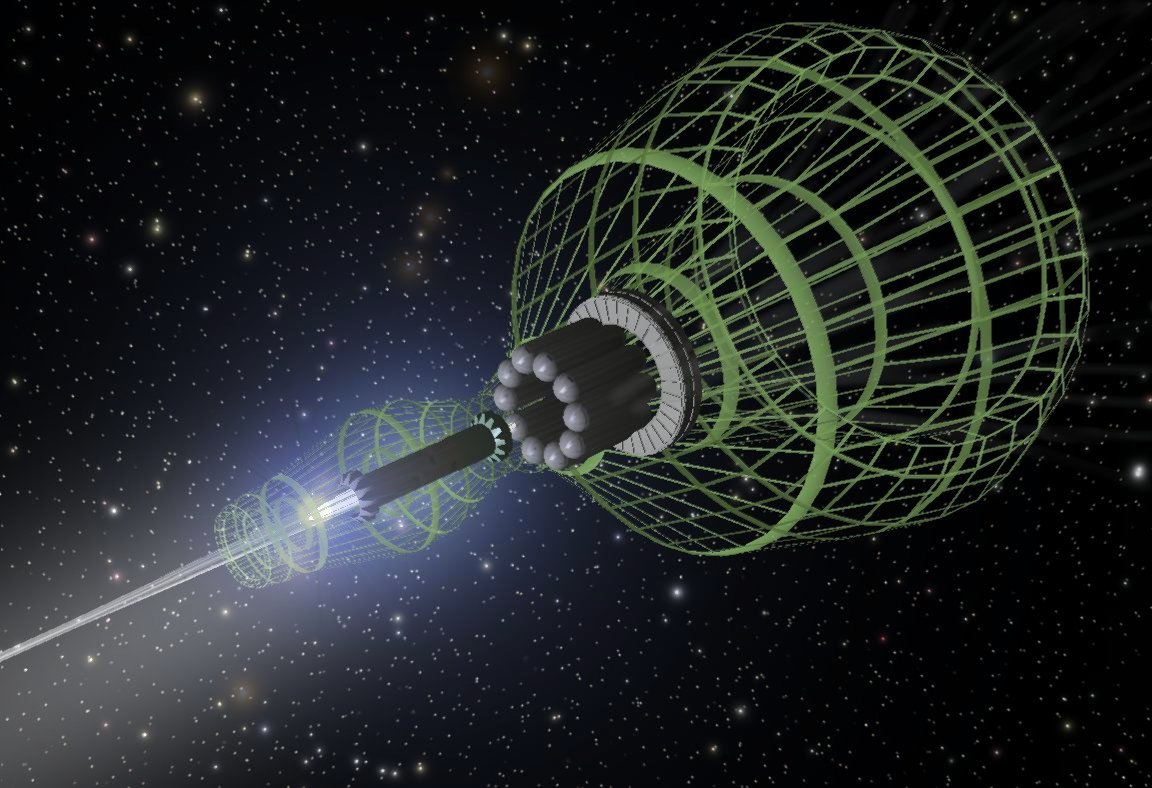My final article dedicated to the memory of Neil Armstrong. The idea is to study quantitatively the relativistic rocket motion with numbers, after all we have deduced the important formulae, and we will explain what is happening in the two frames: S’-frame (in motion), S-frame (in rest on Earth). There are many “variations” of this problem, also called “Langevin’s paradox” or “the Langevin’s interstellar trip” problem by some authors. Here, we will follow the approach suggested in the book Gravitation, by Misner, Wheeler and Thorne, and we will study the interstellar trip (in the frame of special relativity) with the following conditions:

1st. Spacetime is locally minkovskian (i.e., spacetime is flat). The solution we will expose would not be valid in the case of an interstellar trip to a very far away quasar, or a very very long distance (about thousand millions of lightyears) where we should take into account the effect of the expansion of the Universe, i.e., that on large scales, spacetime is curved (in particular, accordingly to the current data, it is pseudoriemannian). Thus, we can use special relativity in order to calculate distances, velocities and accelerations from the purely kinematical sense. After all, it is logical, since General Relativity says that locally, in small enough regions of spacetime, spacetime is described by a minkovskian metric.

2nd. We select a 4 stage accelerated motion with our rocket. We will assume that our rocket is 100% efficient in the sense it uses photons as propellant particles. The four stages are: acceleration from rest to g (acceleration step 1), decceleration to rest with -g until we approach the destination (that would be the one-way trip), acceleration with -g (seen from the S-frame) and decceleration with +g ( with respect to the S-frame) to reach Earth again in rest at the end of the round-way trip. Schematically, we can draw a spacetime sketch of this 4 stage journey:Please, note the symmetry of the procedure and the different travel steps.

3rd. We set g=9.8m/s², or, as we saw in one of our previous posts, so we use g=1.03lyr/yr² (in units where c=1).

4th. We can not refuel during the travel.

5th. In the case we return to Earth, we proceed to come back after we stop at the destination immediately. In this case, there is no refuel of the starship or rocket in any point of the trip.

6th. We neglect any external disturbance which can stop us or even destroy us, e.g., micrometeorites, cosmic radiations, comets, and any other body that could alter our route. Indeed, this kind of stuff has to be seriously considered in any realistic travel, but we want to solve an ideal problem consisting in an ideal interstellar trip according to the current knowledge.

The main quantities we have to compute are:

1. In the S’-frame of the rocket, in years. They are, respectively, the time we are accelerating with +g, the time we are decelerating with -g, and the total time accelerating supposing we return to earth immediately from our destination target.

2. Distance in which we are accelerating (x, in light years), seen from the S-frame (we will not discuss the problem in the S’-frame, since it involves lenght contraction and it is more subtle in the calculations). According to our previous studies, we have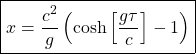3. Maximum depth in space D=2x. This allows us to select our target or destination to set the remaining parameters of the trip. Due to the symmetry of our problem, we have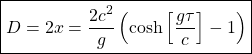4. Total length travelled by the spaceship/rocket (in the S’frame) in the roundtrip ( to the destination and back).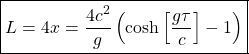5. Maximum speed to an specific destination, after accelerating in a given proper time. It reads:Indeed, the relativistic gamma factor is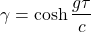6. S-frame duration t(years) of the stage 1 (acceleration phase 1). It is7. One way duration of the trip (according to the S-frame):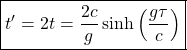8. Total duration of the trip in the S-frame ( round way trip):9. Mass ratio of the final mass with the initial mass of the spaceship after 1 stage, 2 stages ( one way trip) and the 4 stages total round way trip: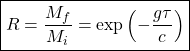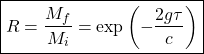10. Fuel mass vs. payload mass ratio (FM/PM) after 1 stage, 2 stages (until the destination) and the total trip: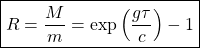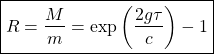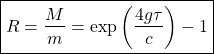We can obtain the following data using these expressions varying the proper time: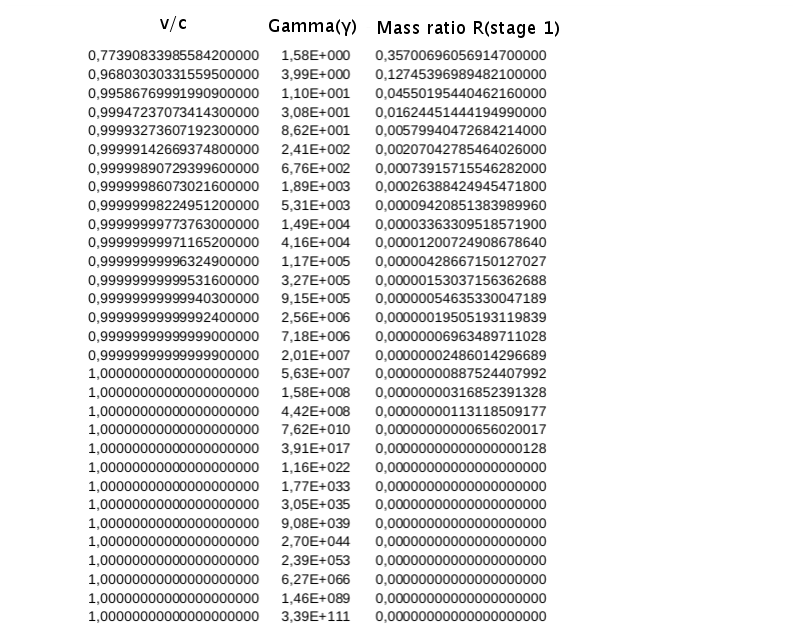Here in the last two entries, data provided to be out of the limits of our calculator ( I used Libre Office to compute them). Now, we can make some final observations:

1st. We can travel virtually everywhere in the observable universe in our timelife using a photon rocket, a priori. However, it shows that the mass-ratio turns it to be theoretically impossible.

2nd. Remember that in the case we select very long distances, these calculations are not valid since we should use General Relativity to take into account the expansion of spacetime.

3rd. Comparewith t,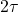with,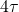with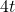. For instance, for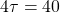then we get 57700 years, and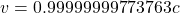, FM/PM=30000 (multiplying por m-kilograms gives the fuel mass).

4th. In SR, the technological problems are associated to the way photon rockets have, the unfavorable mass ratios (of final mass with initial mass) and fuel mass/payload rations necessary in the voyage (supposing of course, current physics and that we can not refuel during the trip).

5th. You can choose some possible distance destinations (D=2x) and work out the different parameters of the travel with the above equations.

In this way, for instance, for some celebrated known space marks, arriving at complete stop ( you can make other assumptions and see how the answer changes varying g or varying the arrival velocity too), we can easily get:

Example 1: 4.3 ly Nearest star (Proxima Centauri) in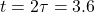yrs (S’-frame).

Example 2: 27 ly Vega (Contact movie and book) star in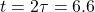yrs (S’-frame).

Example 3: 30000ly, our galactic center, in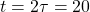yrs (S’-frame).

Example 4: 2000000ly , the Andromeda galaxy, in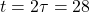yrs(S’-frame).

Example 5: Generally, you can travelly anywhere (neglecting curved spacetime) inyears (S’-frame).

This site uses Akismet to reduce spam. Learn how your comment data is processed.